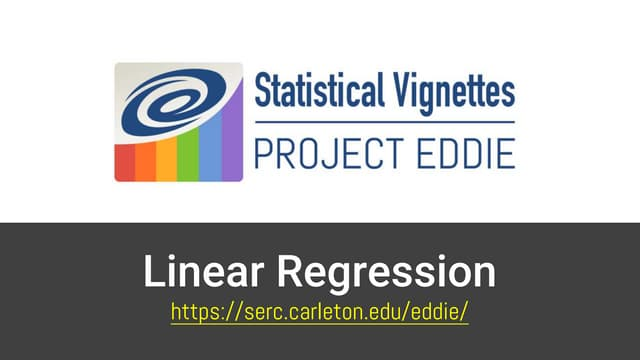# Linear Regression

## Summary

Linear Regression is similar to the correlation coefficient in that both are utilized to understand the relationship between two sets of measurements. Regression expands this concept to create modeled predictions from the extension of the best fit line between a dependent and independent variable. This vignette will help build a student's understanding of linear regression and how to interpret R2 coefficients by analyzing lines of best fit.

## Learning Objectives

• Demonstrate the ability to identify the independent and dependent variables
• Investigate the relationship between two datasets and use the extent to which they vary together to predict future values
• Recognize that not all data relationships can be described using the Correlation Coefficient

## Context for Use

This vignette can be used in either a single lab or lecture session and should take between 15 and 20 minutes for introductory or intermediate level students.

The vignette has been implemented in conjunction with the EDDIE Climate Change Module and utilized in both lab and lecture for the introductory Environmental Science courses at Queens College (CUNY) and at Loyola University of Chicago and is suitable for use as a stand alone activity or as a supplement to where the relationship between two variables is of interest.

## Description and Materials

View the PowerPoint file and the instructor notes to help engage students during the presentation of the vignette.

StatVignette02-Regression.pptx (PowerPoint 2007 (.pptx) 13.4MB Sep21 20)Click to view